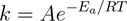The Arrhenius equation is based on the the collision model, and relates the activation energy to the rate constant, k:A: Constant called the frequency factor. It's related to the frequency of collisions, and the orientation of the reacting molecules.

R: The ideal gas constant (8.314 J//mol/K)

T: Temperature (in Kelvin)

Ea: Activation energy (joules or kilojoules per mole)

e: Unitless constant of 2.7183 (base of the natural logarithm)

−Ea/RT represents the fraction of collisions with sufficient energy to produce a reaction

As activation energy increases, the rate constant decreases because the fraction of molecules that possess the required energy is smaller. Therefore, the reaction rate decrease as Ea increases.